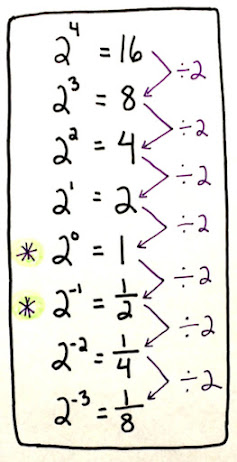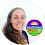## Pages

### Why a zero exponent = 1 (and negative exponents, too)

Why is a number raised to the zero power equal to 1? And why do terms with negative exponents become fractions? Are we able to see this through visual models?

Yes!

In this short video, you'll see how exponents take on a pattern and can be modeled concretely with cut paper. We'll start with 3 raised to the 2nd power and work our way to 3 to the -2.Exponent patterns

Once students start to see the pattern, exponents start to click. Here's a chart I'd write on the board whenever questions arose about zero and negative exponents. Later, we move on to fractional exponents.

Exponents activities

1.My students always seem to struggle with understanding why a number raised to a 0 exponent equals 1. This video and your visual with the exponent patterns is a great way for students to visualize this concept. Thanks for sharing.

2.It stems from the quotient rule for exponents. 2^3/2^3 = 1 but it is also 2^{3-3} = 2^0, so 1= 2^0

3.AnonymousJuly 23, 2023

The image you included or the pattern for 2 with exponents was so perfect. I wish I would have seen it ages ago. This finally clicked!

1.Thank you so much for letting me know this! I'm so glad it helped!

Comment